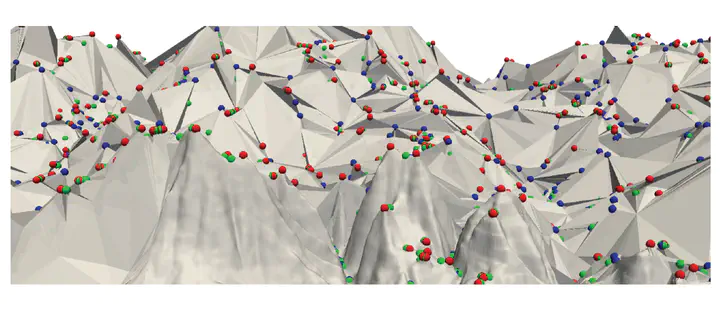# Hierarchical Forman Triangulation: A multiscale model for scalar field analysis### Abstract

We consider the problem of analyzing the topology of scalar fields defined on a triangulated shape by using a multi-scale approach, which allows reducing storage costs and computation times, and supports interactive inspection and classification of topological features. We define and implement a multi-scale model that we call a Hierarchical Forman Triangulation (HFT), where a 3D shape or a terrain is discretized as a triangle mesh, and its topology is described by defining a discrete Morse gradient field based on function values given at the vertices of the mesh. We introduce a new edge contraction operator, which does not change the behavior of the gradient flow and does not create new critical points, and we apply it in combination with a topological simplification operator which eliminates critical elements in pair. By combining the two operators in a sequence, we generate the HFT. We discuss and implement a compact encoding for the HFT that has a lower storage cost with respect to the triangle mesh at full resolution. We show the effectiveness of this new hierarchical model by extracting representations of terrains and shapes endowed with a scalar field at different, uniform and variable, scales and by efficiently computing topological features and segmentations.

Type
Publication
Computers & Graphics